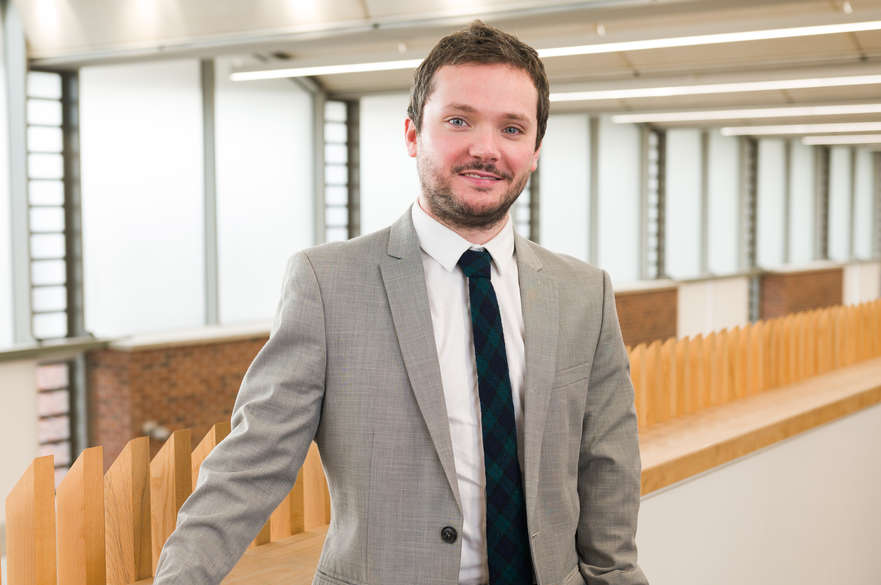# MarkWilkinson

## Senior Lecturer

### Physics and Mathematics

Staff Group(s)
Physics and Mathematics

## Role

Mark Wilkinson is a Senior Lecturer in Mathematics. His research lies in the analysis of partial differential equations and their applications fluid dynamics. He obtained his MSt. in Mathematics from the University of Cambridge (Trinity College) and his doctorate (D.Phil) in Mathematics from the University of Oxford (St. Edmund Hall). He arrived at NTU in February 2020.

For the academic year 2020/21, Mark is module leader for the third-year module "Differential and Integral Equations", and he teaches the second half of the second-year module "Differential Equations and Modelling".

## Research areas

Mark's research interests are in the analysis of partial differential equations (PDE), in particular PDE which come from the applied sciences. His current areas of interest are the semi-geostrophic equations of atmospheric fluid dynamics, the Boltzmann equation and particle dynamics, as well as the Landau-de Gennes theory of liquid crystals. In particular, current work is being carried out in the following areas:

• The Semi-geostrophic Equations - Understanding the dynamics of the troposphere, the lowest layer of the Earth's atmosphere, is crucial in the modelling and prediction of the Earth's weather. Although atmospheric fluid is governed by the Navier-Stokes equations in the presence of rotation, its study can be simplified owing to the fact that it is predominantly flow phenomena on large length scales (of the order of tens of kilometres) which drive its basic motion. In the low Rossby number asymptotics of Navier-Stokes, it is the semi-geostrophic equations (SG) which govern these large length scale phenomena. Together with researchers in Edinburgh, Prof Beatrice Pelloni and Dr David Bourne, and the Met Office (Prof Mike Cullen), I work on the rigorous mathematical analysis of SG, as well as the development of rigorous numerical schemes which compute solutions of this active semi-linear transport equation.
Recent publication(s): https://doi.org/10.1098/rspa.2018.0787
• The Dynamics of Hard Sphere Systems - The energy-preserving dynamics of hard spheres has been actively studied over the past 100 years. It is mathematically rich, and it can be viewed as a classical model for the atomic motion of real-world gases comprised of monatomic particles. Despite its apparent simplicity, there remain many outstanding mathematical problems on the dynamics of hard spheres. I study the dynamics of N hard spheres in space using techniques from the area of mathematical billiards and symplectic geometry. I am particularly interested in the global-in-time Boltzmann-Grad limit of the BBGKY hierarchy associated to N hard sphere systems.
• Kinetic Theory of Non-spherical Particles - The theory of the Boltzmann equation for spherical particles is a very well-developed mathematical theory. However, from a physical perspective, one might deem it to be inappropriate. Indeed, most gases in our everyday experience (such as Oxygen O2 or Hydrogen H2) are not monatomic, and so their particles are not well approximated by spheres which do not rotate. Indeed, it is the interaction between translational modes and rotational modes which makes the dynamics of a gas comprised of non-spherical particles mathematically interesting. I work on the rigorous development of the Boltzmann equation, the canonical kinetic equation for the study of dilute gases, in the context of non-spherical particle systems.
Recent publication(s): https://doi.org/10.1002/cpa.21761

Mark currently supervises 2 PhD students at the Maxwell Institute of Mathematical Sciences in Edinburgh, Ms Stefania Lisai (Sep. 2017 - present) and Mr Charlie Egan (Sep. 2019 - present), both of whom work on the analysis of the semi-geostrophic equations. See Stefania's recent work here and Charlie's recent work here.

## External activity

• Reviewer for journals including the Archive for Rational Mechanics and Analysis, and the Proceedings of the Royal Society of Edinburgh A
• Winner of the Pioneer Award, the Principal's Public Engagement Prize 2018, Heriot-Watt University
• Contributor to the Royal Institution's Mathematics Masterclass Series (2017, 2018 & 2019)